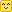Hi!

But maybe you need the solution to another, similar problem that consists in finding the solid angle of a pixel lying in the z=0 plane and orthogonally projected onto the hemisphere, but couldn't find a page with that computation?

Well let me help you with that!First of all, the document that was used initially to do the computations, both in AMD's cube map generator, Rory Driscoll's summary and this page, is the very interesting thesis by Manne Öhrström.

## Cubemap Projection Configuration

The configuration for a cube map is that pixels are lying on a plane z=1 such as $p'=(x,y,1), -1<x<1, -1<y<1$ and we project back onto the unit hemisphere by "normalizing" the vector $p=\frac{\mathbf{p'}}{|\mathbf{p'}|}=\frac{(x,y,1)}{\sqrt{1+x^2+y^2}}$ as shown on the figure below:

The idea is to compute the area of a small element of surface on the hemisphere as we make it vary on the plane, we do that by computing the partial derivatives of $\mathbf{p}$ along x and y that give us the vectors $\frac{\partial \mathbf{p}}{\partial x}$ and $\frac{\partial \mathbf{p}}{\partial y}$.